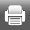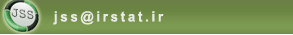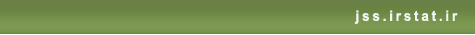[Home ] [Archive]   [ فارسی ]Back to the articles list Back to browse issues page
Bayesian LASSO Regression with Asymmetric Error in High Dimensional
Abstract:   (478 Views)

One of the most critical discussions in regression models is the selection of the optimal model, by identifying critical explanatory variables and negligible variables and more easily express the relationship between the response variable and explanatory variables. Given the limitations of selecting variables in classical methods, such as stepwise selection, it is possible to use penalized regression methods. One of the penalized regression models is the Lasso regression model, in which it is assumed that errors follow a normal distribution. In this paper, we introduce the Bayesian Lasso regression model with an asymmetric distribution error and the high dimensional setting. Then, using the simulation studies and real data analysis, the performance of the proposed modelchr('39')s performance is discussed.

Keywords: Penalized Regression, Bayesian LASSO Regression, Asymmetric Laplace Distribution, Alpha-Skew Normal Distribution, Gibbs Algorithm.
Type of Study: Research | Subject: Statistical Inference